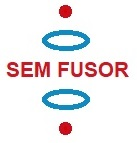A new idea to obtain clean fusion energy

11.9 Confinement of positive ions and electrons with a static electric and magnetic field

Applying magnetic mirror effect to the SEM fusor

Back to main page

Applying an "magnetic mirror" magnetic field, as illustrated in figure 1, we'll get (probably) an extra method to stop the particles escaping in the vertical direction.

So the positive deuterium ions and the electrons in the SEM fusor will be confined both by the static electric field generated by the charged rings and spheres and by the magnetic field. The magnetic field will stop them to escape sidewards, but also (probably) helps in avoiding that they escape in the vertical direction.

Fig. 1. SEM-fusor with magnetic mirror magnetic field.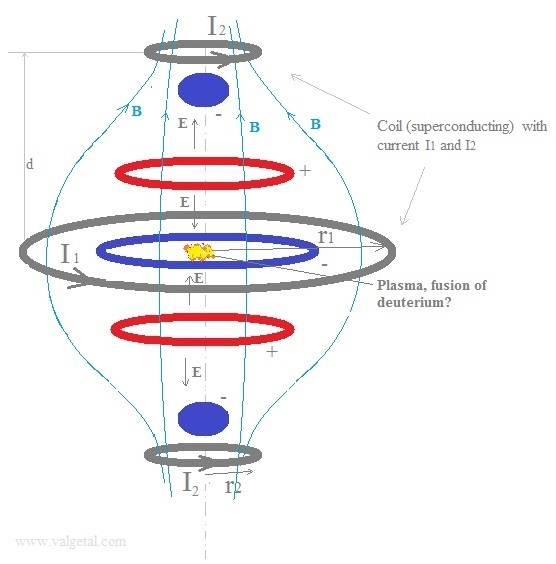In the simulation program we will  input (and vary):
The currents I1 and I2.
The distance d.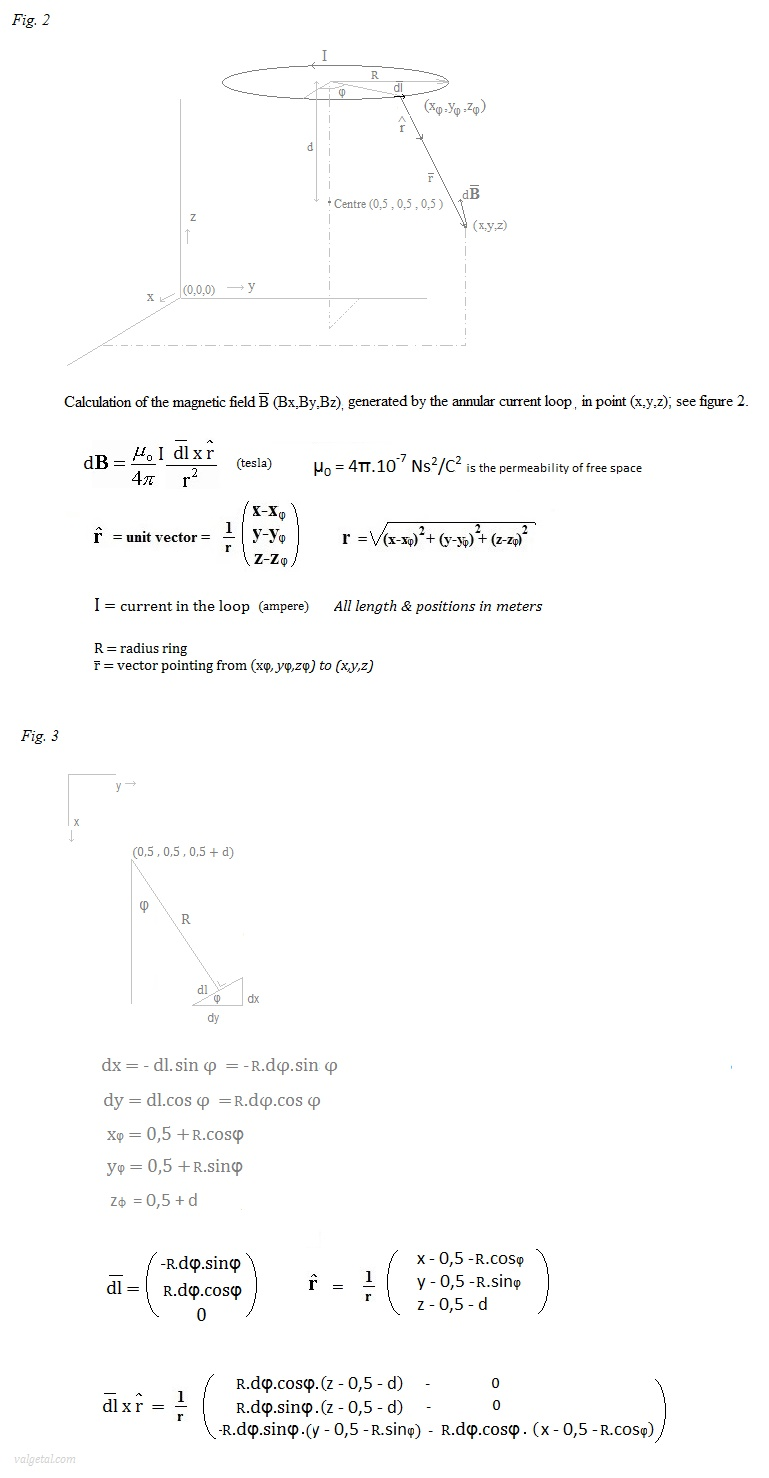In the program we'll take a  φ=1 to 360 loop and calculate with dφ= 2π/360 ->  ∑ dB  in point (x,y,z).
Each dφ= 1
º .

Magnetic Confinement Concepts: a nice animation of the magnetic mirror.

Some experiments:.

 Experiment 11.9.1. Magnetic bottle.Fig.4. Configuration.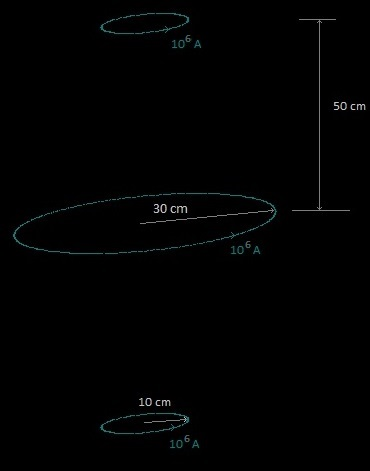The magnetic field is produced by the three current rings as shown in figure 4.  Here only one loop is drawn, but in reality this would be a coil consisting of many loops/strands. The current through the coil (through one strand) would then be less than the stated 106 A. Fig. 5. The magnetic field.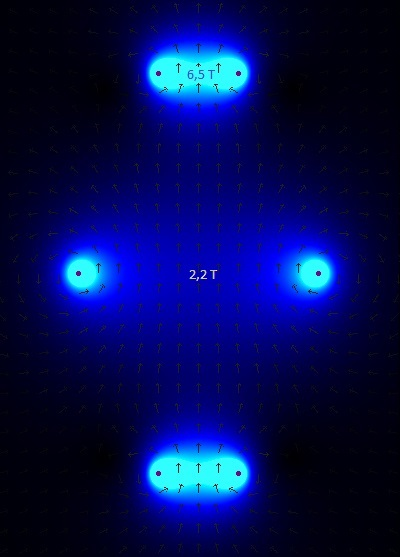The arrows show the direction of the magnetic field, in the plane: x=0,5 mtr (cross section through the centre of the simulation space in a vertical plane, see fig. 2 and  coordinate system ) . The stronger the field, the lighter the colour. In the centre of the rings the magnetic field strength is 2,2 tesla and 6,5 tesla.   Fig. 6. Screenshot of simulation experiment with 20 D+ ions.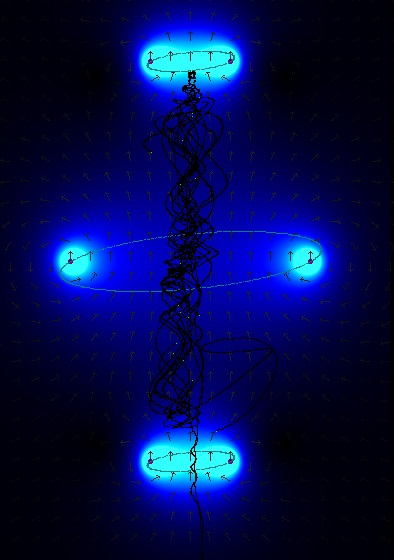20 deuterium ions are generated in the centre region with random speeds up to 3.105 m/s. The majority are trapped in the "magnetic bottle"; a few ions escaped. (dt=10-10 s).   Fig. 7. Screenshot of simulation experiment with 20 D+ ions after  some more elapsed time (2,8 10-5 s).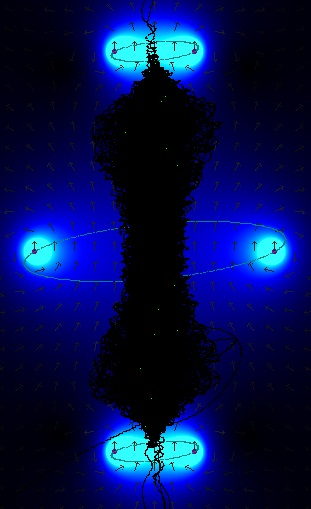Old positions of the ions become black. 13 deuterium ions stayed trapped; 7 ions have escaped. Video of the experiment Conclusion: although the applied magnetic field is quite strong (2,2 T in the middle of the centre ring and 6,5 T in the middle of the other rings), not all deuterium ions kept confined. The ions were generated with random speeds up to 3E6 m/s, the same as in the experiments with the SEM fusor. The simulation program seems to work properly (it shows the magnetic mirror effect). . Experiment 11.9.2.  Helmholtz coil Fig. 8.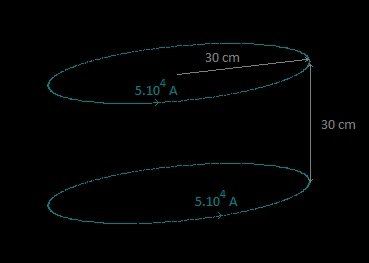The configuration of figure 8. is called Helmholtz coil. It produces a region of nearly uniform magnetic field. Let's see what our simulation program calculates.. Fig. 9. The magnetic field created by the simulation program for the Helmholtz coil of fig. 8.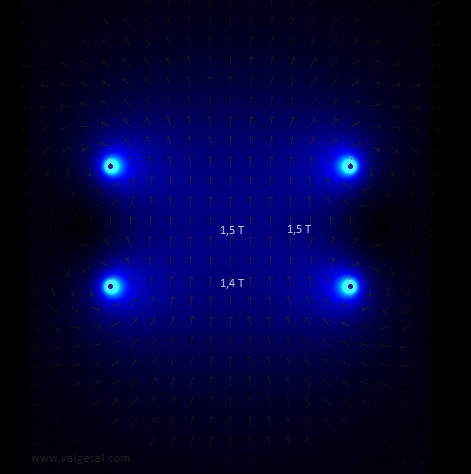The magnetic field at the midpoint between the coils is given by B = (4/5)3/2 .μ0.nI /R  (see μ0 = 4π. 10-7 T.m/A nI = 5.105 A (total current through one coil/current ring) R = 0,30 mtr -> B = 1,4986 T   (the same, rounded to one decimal,  as the simulation program)  Good! . Experiment 11.9.3.  SEM fusor with magnetic mirror fieldSimilar to Experiment 11.14a, but with less voltages, the same initial speed of the particles and more or less the same magnetic field strength, but now in the form of a magnetic mirror. Fig. 10. The configuration of the experiment.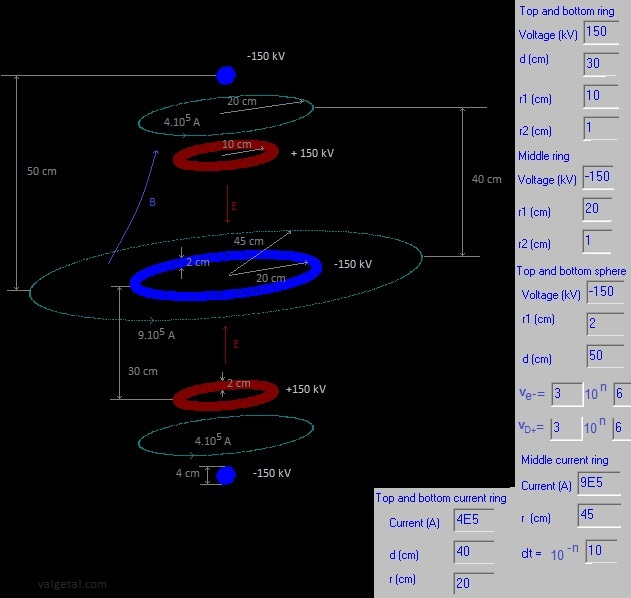. Three charged rings, producing an electric field, and three current rings producing a magnetic mirror field. Fig. 11. Screenshot. In the centre region 20 D+ ions have been generated with random speeds up to 3.106 m/s. Fig. 12. Screenshot with 50 D+ ions, 20 electrons, after 5,9E-5 s.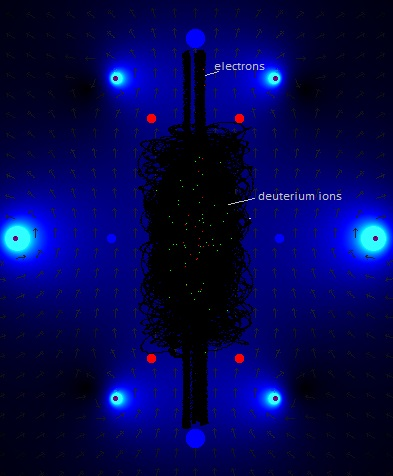The particles stay confined with some less voltages (150 kV instead of 200 kV) as in the similar experiment with a uniform magnetic field. So applying a magnetic mirror field seems to improve (a little bit) our SEM fusor (but it's not a panacea..). . Experiment 11.9.4.  SEM fusor with reversed polarities and with a constant magnetic field Fig. 13.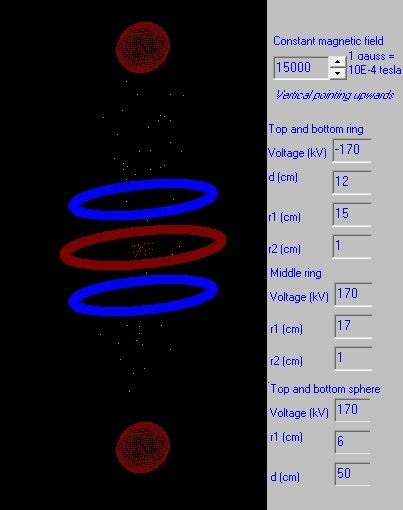We'll try out the configuration of fig. 13. Fig. 14. Screenshot. The arrows indicate the direction of the electric field; the red colour its strength.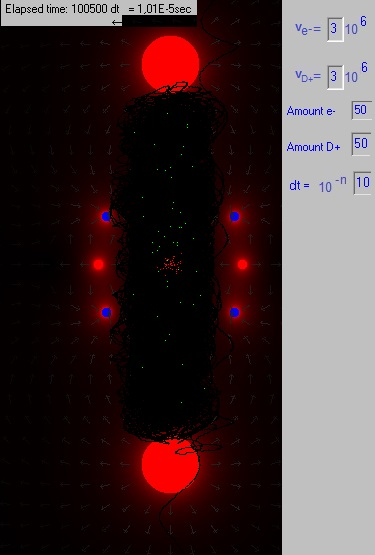Two deuterium ions escaped. The applied voltages should be a little bit higher, and the radius of the charged rings a little bit larger. But, as mentioned already elsewhere, the electrons stay well confined in the centre and could form a virtual cathode, which would attract the D+ ions and be an extra advantage for our SEM fusor. . Experiment 11.9.5.  SEM fusor with reversed polarities and a mirror magnetic field The dimensions are the same as in exp. 11.9.4, the voltages a little bit less (150 kV instead of 170 kV), the magnetic field has more or less the same strength, only it's now not uniform but has the form of a magnetic bottle (mirror). Fig. 15. Screenshot. With this magnetic mirror field the particles stay somewhat better confined as with the uniform magnetic field of exp. 11.9.4. . Experiment 11.9.6.  SEM fusor with reversed polarities, a mirror magnetic field and reduced voltagesFig. 16.  Dimensions Screenshot By applying the magnetic mirror field it seems to be possible to reduce further the applied voltages to +/- 100 kV. But having the current loops so nearby the charges will in reality not be very easy... Experiment 11.9.7. Two rings, magnetic mirror field, particles injected from below to upFig. 17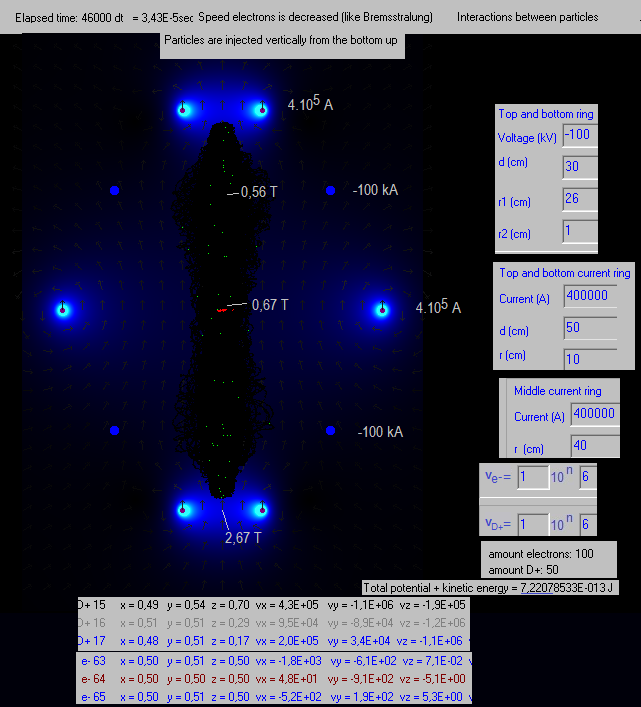Sem Fusor with only two negatively charged rings and a magnetic field in the form of a bottle, generated by three curring carrying loops. The D+ ions are injected just under the bottom ring, and the electrons just above the bottom ring. In a few points the strenght of the magnetic field is indicated. The total potential + kinetic energy stays constant in 3 decimals, which is an indication that the simulation program is rather correct. The speeds of the electrons is decreased in the program, to simulate Bremsstrahlung:  if (vx>1E1) and (vy>1E1) and (vz>1E1) then Begin vx:=vx*0.90; vy:=vy*0.90; vz:=vz*0.90; end; The D+ ions are a little squezed in the centre region, which is a little advantage.28 January 2018      by  Rinze Joustra        www.valgetal.com